Test: Firing Circuits

# Test: Firing Circuits

Test Description

## 30 Questions MCQ Test Power Electronics | Test: Firing Circuits

Test: Firing Circuits for Electrical Engineering (EE) 2023 is part of Power Electronics preparation. The Test: Firing Circuits questions and answers have been prepared according to the Electrical Engineering (EE) exam syllabus.The Test: Firing Circuits MCQs are made for Electrical Engineering (EE) 2023 Exam. Find important definitions, questions, notes, meanings, examples, exercises, MCQs and online tests for Test: Firing Circuits below.
Solutions of Test: Firing Circuits questions in English are available as part of our Power Electronics for Electrical Engineering (EE) & Test: Firing Circuits solutions in Hindi for Power Electronics course. Download more important topics, notes, lectures and mock test series for Electrical Engineering (EE) Exam by signing up for free. Attempt Test: Firing Circuits | 30 questions in 30 minutes | Mock test for Electrical Engineering (EE) preparation | Free important questions MCQ to study Power Electronics for Electrical Engineering (EE) Exam | Download free PDF with solutions
 1 Crore+ students have signed up on EduRev. Have you?
Test: Firing Circuits - Question 1

### In a single pulse semi-converter using two SCRs, the triggering circuit must produce

Detailed Solution for Test: Firing Circuits - Question 1

A single phase semi-converter has only two SCRs & two diodes. Hence, only two pulses are required in each cycle, one in each half.

Test: Firing Circuits - Question 2

### In a 3-phase full converter using six SCRs, gating circuit must provide

Detailed Solution for Test: Firing Circuits - Question 2

60° x 6(devices) = 360°.

Test: Firing Circuits - Question 3

### In the complete firing circuit, the driver circuit consists of

Detailed Solution for Test: Firing Circuits - Question 3

The driver circuit consists of a pulse amplifier to increase the magnitude of the gate pulse to a sufficient value. The pulse transformer then provides pulses to individual SCRs.

Test: Firing Circuits - Question 4

Find the average gate power dissipation (Pgav) when the maximum allowable gate power dissipation (Pgm) = 10 kW, with a duty cycle = 50 %.

Detailed Solution for Test: Firing Circuits - Question 4

(Pgm) = (Pgav)/Duty Cycle.

Test: Firing Circuits - Question 5

The magnitude of gate voltage and gate current for triggering an SCR is

Detailed Solution for Test: Firing Circuits - Question 5

Higher the temperature lesser will be the gate current required as the temperature must have already excited some of the atoms.

Test: Firing Circuits - Question 6

Find the amplitude of the gate current pulse, when the gate-cathode curve is given by the relation Vg = [(1+10) x Ig] The peak gate drive power is 5 Watts.

Detailed Solution for Test: Firing Circuits - Question 6

(1+10 Ig).Ig = 5 Watts
Ig = 0.59 A.

Test: Firing Circuits - Question 7

The gate-cathode curve for an SCR is given by the relation Vg = (1+10)Ig. The gate voltage source is a rectangular pulse of peak value 15 V and current = 0.659 A. Find the source resistance.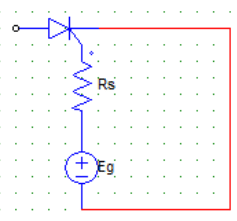Detailed Solution for Test: Firing Circuits - Question 7

Es = Rs.Ig + Vg
Vg = 1+10 Ig
Therefore Rs = (15-1)/0.659.

Test: Firing Circuits - Question 8

Find the triggering frequency when the average gate power dissipation = 0.3 W and the peak gate drive power is 5 Watts. The gate source has a pulse width of 20 μsec duration.

Detailed Solution for Test: Firing Circuits - Question 8

(Pgm) = (Pgav)/Duty Cycle
Duty Cycle = f x T = (Pgav)/(Pgm)
Duty Cycle = 0.3/5
T = 20 μsec.
0.3/5 = f x T
f = (0.3)/(5 x 20 x 10-6) = 3000 Hz.

Test: Firing Circuits - Question 9

The duty cycle can be written as

Detailed Solution for Test: Firing Circuits - Question 9

The duty cycle is defined as the ratio of pulse-on period to periodic time of pulse.
The pulse on period is T, and the periodic time is 1/f.
It is to be noted that T = pulse width whereas f = (1/T1) = frequency of firing or pulse repetition rate.

Test: Firing Circuits - Question 10

The major function of the pulse transformer is to

Detailed Solution for Test: Firing Circuits - Question 10

Isolation of the two circuit is done by the transformer, as the transformer is a magnetically coupled device and any mishap at the load side will not damage the other side of the circuitry.

Test: Firing Circuits - Question 11

In a resistance firing circuit the firing angle

Detailed Solution for Test: Firing Circuits - Question 11

The R firing circuits cannot be used for alpha greater than 90 degrees.

Test: Firing Circuits - Question 12

For a R firing circuit, the maximum value of source voltage is 100 V. Find the resistance to be inserted to limit the gate current to 2 A.

Detailed Solution for Test: Firing Circuits - Question 12

R = 100/2 = 50 Ohm.

Test: Firing Circuits - Question 13

The diode in the R firing circuit

Detailed Solution for Test: Firing Circuits - Question 13

The diode is placed between the resistances and gate which ensures that the current flows in one direction only.

Test: Firing Circuits - Question 14

In case of an RC half wave triggering circuit, the firing angle can be ideally varied between

Detailed Solution for Test: Firing Circuits - Question 14

Unlike the R firing circuit, the RC firing circuits can be used to obtain firing angle greater than 180. Although practically 0 and 180 degree is improbable.

Test: Firing Circuits - Question 15

In the figure given below, the resistance R1 is used to​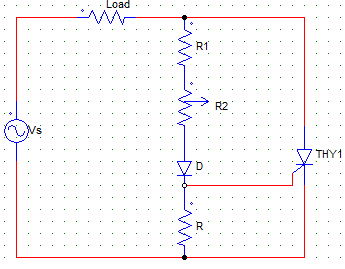Detailed Solution for Test: Firing Circuits - Question 15

As R2 is the variable, R1 makes sure that the current does not exceed the maximum value when R2 is kept at zero position.

Test: Firing Circuits - Question 16

In case of a R firing with R2 as the variable resistance, Vgp (peak of gate voltage) and Vgt(gate triggering voltage) the value of R2 is so adjusted such that

Detailed Solution for Test: Firing Circuits - Question 16

For turning on the device, the peak of gate voltage must be equal to the gate triggering voltage.

Test: Firing Circuits - Question 17

In case of a R firing circuit with Vgp > Vgt

Detailed Solution for Test: Firing Circuits - Question 17

For the values of Vgp great than the gate triggering voltage the firing angle is less than 90°. And for Vgp = Vgt the firing angle is equal to 90°. Α cannot go beyond 90° in case of a R firing circuit.

Test: Firing Circuits - Question 18

The figure shown below is that of a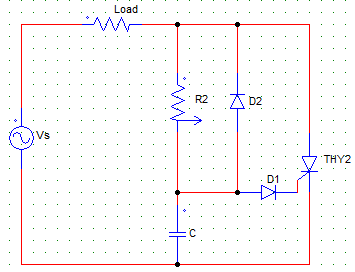Detailed Solution for Test: Firing Circuits - Question 18

The given circuit is a RC half-wave firing circuit.

Test: Firing Circuits - Question 19

The figure shown below is that of an RC firing circuit.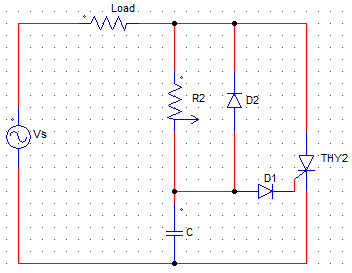In case of negative cycle at Vs, the capacitor C

Detailed Solution for Test: Firing Circuits - Question 19

The current flows through Vs+ – C – D2 – Load – Vs.

Test: Firing Circuits - Question 20

Find the value of R in case of an RC firing circuit which is to be turned on with a source voltage of 150 V and the following parameters.
Igt = 2A
Vd = 1.5V
Vgt = 125 V

Detailed Solution for Test: Firing Circuits - Question 20

R = (Vs-Vgt-Vd)/Igt.

Test: Firing Circuits - Question 21

For the following RC triggering circuit with R load and a firing angle of α, the voltage across the R load is zero for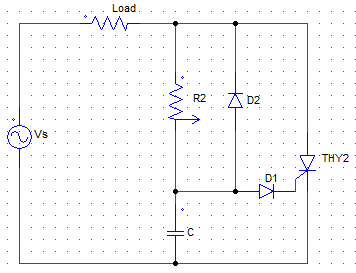Detailed Solution for Test: Firing Circuits - Question 21

The SCR is triggered at α therefore voltage appears across the load from ωt = α to π only as in case of a half wave firing circuit the SCR is naturally commuted at π.

Test: Firing Circuits - Question 22

For the following circuit, the SCR (thy2) is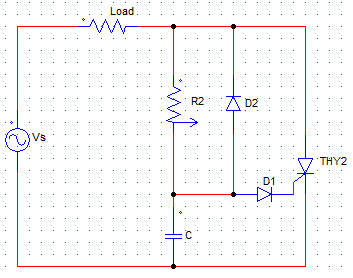Detailed Solution for Test: Firing Circuits - Question 22

Circuit is that of a half-wave RC firing circuit where natural commutation takes place at π due to the sinusoidal voltage source.

Test: Firing Circuits - Question 23

For the following RC triggering circuit with R load and a firing angle of α, the voltage across the SCR (thy2) will be zero for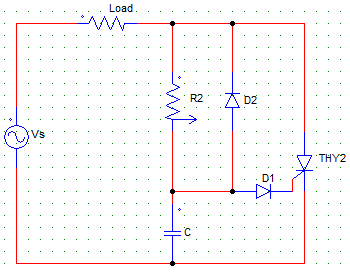Detailed Solution for Test: Firing Circuits - Question 23

The SCR is triggered at α therefore voltage appears across the load from ωt = α to π only as in case of a half wave firing circuit the SCR is naturally commuted at π.

Test: Firing Circuits - Question 24

In case of an RC full wave firing circuit with R load, the voltage across the load is zero for____________

Detailed Solution for Test: Firing Circuits - Question 24

The SCR is not triggered(turned-on) until α, hence no current flows until α.

Test: Firing Circuits - Question 25

For an RC full wave firing circuit the empirical formula for calculating the value of RC is

Detailed Solution for Test: Firing Circuits - Question 25

RC = 157/ω, Where ω is the angular frequency.

Test: Firing Circuits - Question 26

Pulse triggering can be only used by the _____________ type of triggering circuit

Detailed Solution for Test: Firing Circuits - Question 26

R & RC produce prolonged pulses which increases the gate power dissipation. There is no RLC firing circuit.

Test: Firing Circuits - Question 27

The UJT terminals are

Detailed Solution for Test: Firing Circuits - Question 27

The terminals are Emitter, Base1 & Base 2.

Test: Firing Circuits - Question 28

In case of the UJT firing circuit, when the UJT turns on

Detailed Solution for Test: Firing Circuits - Question 28

When the UJT is fired, C starts to discharge.

Test: Firing Circuits - Question 29

In the UJT firing circuit, the pulses are generated while the

Detailed Solution for Test: Firing Circuits - Question 29

When the C starts to discharge through the resistance with time constant RC the UJT turns on & hence pulses are generated.

Test: Firing Circuits - Question 30

Find the value of the charging resistor in case of a UJT firing circuit with firing frequency of 2 kHz, C = 0.04 μF, η = 0.72

Detailed Solution for Test: Firing Circuits - Question 30

T = 1/f = 1/2 kHz
R = T/C ln(1/1-η).

## Power Electronics

5 videos|39 docs|63 tests
 Use Code STAYHOME200 and get INR 200 additional OFF Use Coupon Code
Information about Test: Firing Circuits Page
In this test you can find the Exam questions for Test: Firing Circuits solved & explained in the simplest way possible. Besides giving Questions and answers for Test: Firing Circuits, EduRev gives you an ample number of Online tests for practice

## Power Electronics

5 videos|39 docs|63 tests